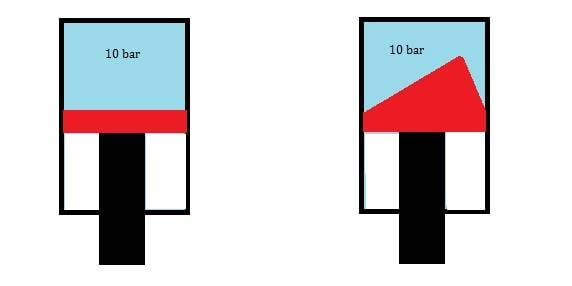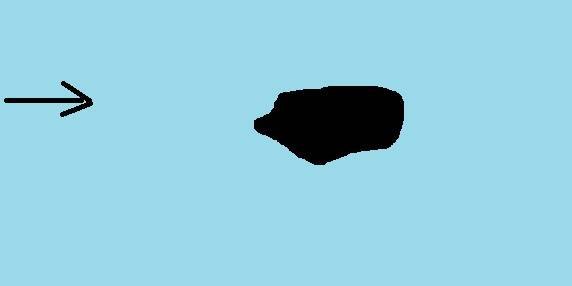# Why constant pressure over different shapes produces the same force?

John Mcrain
Left and right piston produce same vertical force.On both piston, side force is zero,how this is possible if left piston don't has simetrical shape?

Why shape of object is irrelevant when fluid has constant/same pressure around object?
How can I prove with integration of pressure over left piston surface that side force is zero?•Delta2

Mentor
Left and right piston produce same vertical force. On both piston, side force is zero,how this is possible if left piston don't has simetrical shape?

Why shape of object is irrelevant when fluid has constant/same pressure around object?
How can I prove with integration of pressure over left piston surface that side force is zero?
It seems like you already know the answer, but just want to see the math. Start with an easy shape and just do the integration with trig and some simple arithmetic. For example, if the piston shape is a 30 degree incline, the horizontal width is still 1, and the angled face is 1.15. The vertical component of force is still 1, the horizontal is 0.5 and the total is 1.15.

•Lnewqban
John Mcrain
It seems like you already know the answer, but just want to see the math. Start with an easy shape and just do the integration with trig and some simple arithmetic. For example, if the piston shape is a 30 degree incline, the horizontal width is still 1, and the angled face is 1.15. The vertical component of force is still 1, the horizontal is 0.5 and the total is 1.15.
What if I have object in flow where shape is not symetric and different pressures at each point ,how than I integrate total force?What if I have object in flow where shape is not symetric and different pressures at each point ,how than I integrate total force?
It is just vector calculus; with a pressure ##p = -\rho g z##, defined up to addition of an arbitrary constant (##p' = p + a \implies \nabla p = \nabla p'##) the surface force is$$\mathbf{F} = -\oint_{\partial \Omega} p \, d\mathbf{S} = -\int_{\Omega} \nabla p \, d\tau = \rho g \mathbf{z} \int_{\Omega} d\tau = \rho g V \mathbf{z}$$where ##\rho## is here the fluid density, and ##\mathbf{z}## a unit vector pointing vertically upward [this assuming constant density of fluid!].

There is also a heuristic 'physicsy' explanation. Imagine that you replace the body with a parcel of fluid of the same shape; it is clear that this parcel of fluid must be in equilibrium, and since its weight is ##-\rho g V \mathbf{z}##, the buoyant force (the total force due to the surface pressure forces, which depends on the shape of the boundary) must equal ##\rho g V \mathbf{z}##.

Last edited by a moderator:
•Delta2, vanhees71, Dale and 1 other person
Mentor
What if I have object in flow where shape is not symetric and different pressures at each point ,how than I integrate total force?
You need an equation to describe the shape, or if is a real object a method for measuring it.

John Mcrain
You need an equation to describe the shape, or if is a real object a method for measuring it.
Do you integrate pressures normal to the surface object or component of pressure which is normal to the chord line?

Mentor
Do you integrate pressures normal to the surface object or component of pressure which is normal to the chord line?
Parallel to the chord line*. This is a different problem than the one you started with, but remember, you were looking for pressure applied over a cross section. It's the cross sectional area that you are looking for here. But note, as this is now a fluid dynamics problem, the pressure is no longer going to be a constant.

Is there a reason why you changed the scenario so drastically? Is there a direction to this line of questioning?

 *There's a hidden assumption in there about what you are asking, so I can't be certain if parallel or perpendicular. You will have to tell us what you are trying to examine.

John Mcrain
Parallel to the chord line. This is a different problem than the one you started with, but remember, you were looking for pressure applied over a cross section. It's the cross sectional area that you are looking for here. But note, as this is now a fluid dynamics problem, the pressure is no longer going to be a constant.

Is there a reason why you changed the scenario so drastically? Is there a direction to this line of questioning?
from post 3 i am talking about dynamic problems

Mentor
from post 3 i am talking about dynamic problems
What about dynamic problems? What is it you are trying to find/what problem are you trying to solve?

John Mcrain
What about dynamic problems? What is it you are trying to find/what problem are you trying to solve?
I don't understand how pressure is intergrated over object...procedure from start to the end..

Mentor
Let me try this again:
What if I have object in flow where shape is not symetric and different pressures at each point ,how than I integrate total force?
For this problem, you appear to be asking for the total aerodynamic/hydrodynamic force. Yes, that's integration over the entire surface to find a single resultant vector.

Mentor
By the way, do you see how it could be jarring to the reader/person trying to help, that you made such a massive jump in complexity level of the problem, with zero explanation?

•Dale
John Mcrain
By the way, do you see how it could be jarring to the reader/person trying to help, that you made such a massive jump in complexity level of the problem, with zero explanation?
I didnt aks to solve Navier Stokes equation..
If I know pressure distribution around object how then is proccedure to set integration to find total force.

Mentor
I didnt aks to solve Navier Stokes equation..
If I know pressure distribution around object how then is proccedure to set integration to find total force.
There's no possible way that you could know the pressure distribution, so we have to stop there.

Arjan82
What if I have object in flow where shape is not symetric and different pressures at each point ,how than I integrate total force?

View attachment 275692

For your original post the there is indeed no net side force. For the picture you are showing here (rocky thing in free stream?) the side force is not necessarily zero.

Integrating the force over an object is obviously just computing this integral (cartesian coordinates):
$$F_i = \oint_{\Omega} \left(p n_i + \tau_i\right) dS$$

Which is a contour integral around the whole surface area where ##\Omega## is the boundary of the volume (i.e. its surface, in 2D a line), ##i## refers to the component of the resultant force (##x##, ##y## or ##z##), ##p## is the pressure, ##n_i## is the ##i^{th}## component of the surface normal, ##\tau_i## is the ##i^{th}## component of the frictional (tangential) force on the surface and ##dS## is a small part of the surface (line in 2D). The result is ##F_i##, which is the total force in the ##i^{th}## direction.

Obviously you need to know the pressure and frictional distribution over the surface first to be able to solve this. If you don't know how to solve such an integral, please grab a math book or make a very specific example and try to work it out yourself. We'll help with the missing bits.

•Delta2, vanhees71 and russ_watters
John Mcrain
There's no possible way that you could know the pressure distribution, so we have to stop there.
imagine that I meassured static pressure around object..

Mentor
imagine that I meassured static pressure around object..
@Arjan82's answer is a good one.

•